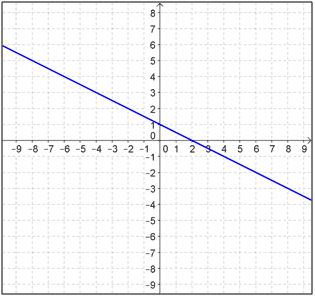# Identifying the slope of a line

• Kirito123

## Homework Statement

identifying the slope of the line?[/B]y2-y1/x2-x1

## The Attempt at a Solution

i chose two points on the line, the first point is (8,-3) and the second point is (-6,4). Are these points accurate?
i used the slope equation to solve for the slope of the line from the graph:
4-(-3) / -6-8 = -7/14 = -1/2

is this right?

Well done. One tip: use brackets -- not 4-(-3) / -6-8 but ( 4-(-3) ) / ( -6-8 )

can the answer also be 7/-14 and 1/-2

## Homework Statement

identifying the slope of the line?[/B]
View attachment 99414

y2-y1/x2-x1

## The Attempt at a Solution

i chose two points on the line, the first point is (8,-3) and the second point is (-6,4). Are these points accurate?
i used the slope equation to solve for the slope of the line from the graph:
4-(-3) / -6-8 = -7/14 = -1/2

is this right?

What you wrote means ##y_2 - \frac{y_1}{x_2} - x_2##, which is not correct. Did you mean ##\frac{y_2 - y_1}{x_2 - x_1}##? If so, you need to use parentheses, like this: (y2-y1)/(x2-x1).

Both of these are the same value as ##-{1\over 2}##, which is the simplest way to express that value. Whatever points you choose, you always get ## - {a\over 2a}## as a result.

got it, thanks!

i chose two points on the line, the first point is (8,-3) and the second point is (-6,4). Are these points accurate?
i used the slope equation to solve for the slope of the line from the graph:
4-(-3) / -6-8 = -7/14 = -1/2

is this right?

its a straight line ,so where lies the confusion about its being wrong?

i solved this question previously and got a different slope compared to what I'm getting now. Then i realized that my points were wrong when doing it the first time, so i wanted to make sure the points i chose were correct in my second attempt.

•drvrm